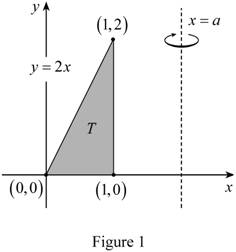# To express: The limit ( a ) in terms of volume of solid ( V ) using the method of cylindrical shell.### Single Variable Calculus: Concepts...

4th Edition
James Stewart
Publisher: Cengage Learning
ISBN: 9781337687805### Single Variable Calculus: Concepts...

4th Edition
James Stewart
Publisher: Cengage Learning
ISBN: 9781337687805

#### Solutions

Chapter 6.3, Problem 34E
To determine

## To express: The limit (a) in terms of volume of solid (V) using the method of cylindrical shell.

Expert Solution

The expression of the limit (a) in terms of V is 0.159V+0.6667_.

### Explanation of Solution

Given:

The co-ordinates of the triangular region (x,y) are (0,0),(1,0), and (1,2).

The equations are y=2x, x=a; where a>1.

Calculation:

Plot a graph using the co-ordinates of the triangular region (x,y) are (0,0),(1,0), and (1,2):

Draw the shell as shown in Figure 1.Calculate the volume using the method of cylindrical shell:

V=ab2πx[f(x)]dx (1)

Substitute 0 for a, 1 for b, (ax) for x, and 2x for [f(x)] in Equation (1).

V=012π(ax)(2x)dx=4π01(axx2)dx (2)

Integrate Equation (2).

V=4π[a(x1+11+1)(x2+12+1)]01=4π[a2x213x3]01=4π[(a×122133)0]

=4π(0.5a13)V=6.283a4.18879 (3)

Rearrange Equation (3).

6.283a=V+4.18879a=V+4.188796.283=0.159V+0.6667

Hence, the expression of the limit (a) in terms of V is 0.159V+0.6667_.

### Have a homework question?

Subscribe to bartleby learn! Ask subject matter experts 30 homework questions each month. Plus, you’ll have access to millions of step-by-step textbook answers!